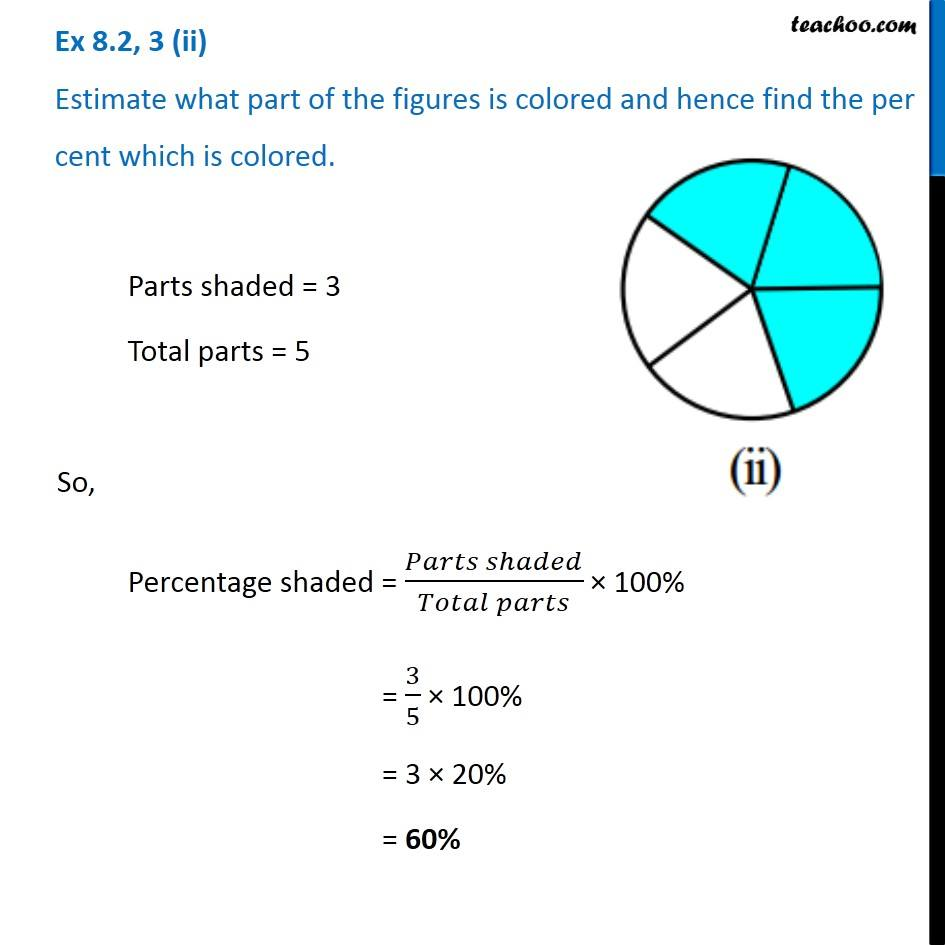1. Chapter 8 Class 7 Comparing Quantities
2. Serial order wise
3. Ex 8.2

Transcript

Ex 8.2, 3 (ii) Estimate what part of the figures is colored and hence find the per cent which is colored. Parts shaded = 3 Total parts = 5 So, Percentage shaded = (𝑃𝑎𝑟𝑡𝑠 𝑠ℎ𝑎𝑑𝑒𝑑)/(𝑇𝑜𝑡𝑎𝑙 𝑝𝑎𝑟𝑡𝑠) × 100% = 3/5 × 100% = 3 × 20% = 60%

Ex 8.2

Chapter 8 Class 7 Comparing Quantities
Serial order wise